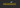# The difference between Rest and spread operator in JavaScriptIn this tutorial, we will learn the difference between rest and spread operator in JavaScript.

## Rest Parameters ( … )

Rest parameters help us to pass an infinite number of function arguments.

Example:

``````function sum(a,b,...remaining){
console.log(a);
console.log(b);
console.log(remaining);
}

sum(1,2,3,4,5,6,7);

//a -> 1

//b - > 2

//remaining - > [3,4,5,6,7]``````

In the above code, the `sum` function accepts three arguments `a`, `b` and `remaining`.where the first two arguments values are `1`, `2` everything we passed after is represented as an array `[3,4,5,6,7]`.

Spread operator helps us to expand the strings or array literals or object literals.

• Splitting the strings
`````` let name = "JavaScript";

let arrayOfStrings = [...name];

console.log(arrayOfStrings);

// Ouptut -> ["J", "a", "v", "a", "S", "c", "r", "i", "p", "t"]``````

In the above example, we are using `...` spread operator to split the single string into an array of strings.

• Merging arrays
``````const group1 = [1,2,3];
const group2 = [4,5,6];

const allGroups = [...group1,...group2];

console.log(allGroups)

//output -> [1, 2, 3, 4, 5, 6]``````

In the above code, we combined `group1` and `group2` by using the spread operator.

• Merging Objects
``````const obj1 = {
a: 1,
b: 2
}

const obj2 = {
c: 3,
d: 4
}

const merge  = {...obj1, ...obj2};

console.log(merge); // {a:1, b:2, c:3, d:4}``````

## Using Spread operator in Function calls

We can use the spread operator to pass an array of numbers as a individual function arguments.

``````function sum(a,b,c){
return a+b+c;
}

const nums = [1,2,3];

//function calling

sum(...nums) // 6``````

## Summary

• If you see (…) dots on the function call then it is a spread operator.
• If you see (…) dots on the function parameter then it is a rest parameter.
• Spread operator helps us to merge Arrays or Objects.

## Top Udemy Courses##### JavaScript - The Complete Guide 2020 (Beginner + Advanced)
45,614 students enrolled
52 hours of video content
View Course##### React - The Complete Guide (incl Hooks, React Router, Redux)
284,472 students enrolled
40 hours of video content
View Course##### Vue JS 2 - The Complete Guide (incl. Vue Router & Vuex)
130,921 students enrolled
21 hours of video content
View Course